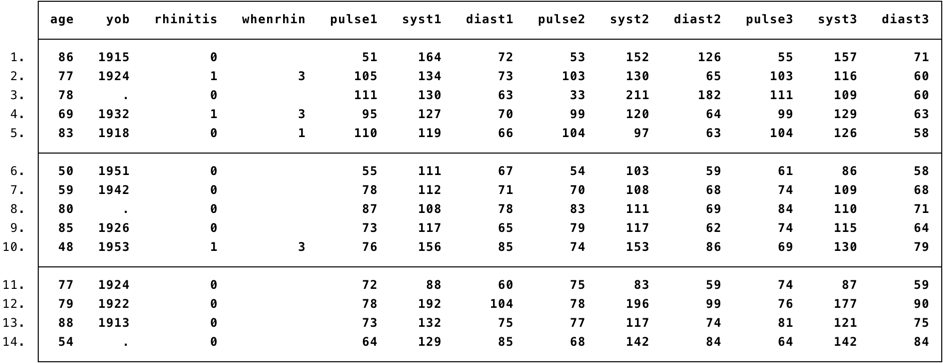﻿ Chapter 2: Introducton to the research process using STATA

# Chapter 2: Introducton to the research process using STATA

### Figure 2.1 and Figure 2.3Figure 2.1 Portion of a dataset showing data collected for particiapnts in a screening survey (example)Figure 2.3 Checking for errors in the data (example)

### Figure 2.1 and Figure 2.3 Code### Figure 2.4### Figure 2.4 Code